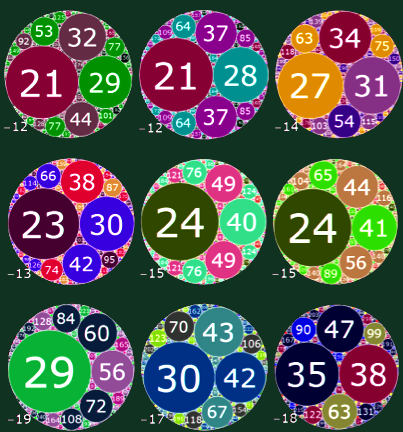## Saturday, June 19, 2010

### Descartes' theorem on kissing circles

Ed Pegg Jr wrote a fun mathematical article (equipped with a Mathematica demonstration) on the Wolfram blog,
The circles of Descartes
The basic topic may be seen on this picture:If you choose three radii of circles, you may always arrange the circles so that they kiss i.e. touch. But if you want to add another, fourth circle that touches each of the previous three as well - which is a special case of the problem of Apollonius, invented about 200 BC - only two possible radii may work. You may either add a nice circle at the center or a big circle that surrounds the three.

(The problem of Apollonius is more general because the three circles you are given as a definition of your exercise don't have to kiss each other. Consequently, there are usually many more solutions than two - but it is still a finite number.)

On the picture above, the numbers are the curvatures i.e. inverse radii (also known as the "bends"). Look at the last circle, one in the lower right corner. You will find four circles of inverse radii +35, +38, +47, -18. The negative sign has to be added to the circle that contains the other three, if any.

You may check that
(35 + 38 + 47 - 18)2 = 10404
2 (352 + 382 + 472 + 182) = 10404
They are equal! More generally,
(k1+k2+k3+k4)2 = 2(k12+k22+k32+k42)
It's not shocking that you may find many integer-valued solutions to the equation. It is somewhat more nontrivial that with the collections on the pictures - and many others - you may actually keep on adding new circles (and construct the so-called Appolonian gasket, the oldest known popular fractal) and the inverse radii stay integer-valued. You may add new circles by replacing an "old 4th circle" by the new one; the corresponding inverse radius is the second solution to a quadratic equation with simple coefficients which is why it remains integer-valued.

René Descartes sent his observation about the formula above to Queen Elizabeth of Bohemia. To be sure, here in Bohemia, most of us don't even realize that she has been a Bohemian (i.e. essentially Czech) queen. Instead, we call her "Alžběta Stuartovna" - a fully English-sounding name.

Because she had an attractive occupation and both people were born in 1596, you may figure out that he had a crush on her. It's always about sex, isn't it? However, the actual reason may have been more political in character. Cartesian philosophy came under attack in the Netherlands around 1643 - when they were 47 years old - and that's when he began his correspondence with the queen. ;-)

Frederick Soddy who received the 1921 chemistry Nobel prize for some work on isotopes is actually more well-known for a 1936 article in Nature that describes similar theorems in the format of a poem. It begins as follows:
“The Kiss Precise”
by Frederick Soddy

For pairs of lips to kiss maybe
Involves no trigonometry.
‘Tis not so when four circles kiss
Each one the other three.
To bring this off the four must be
As three in one or one in three.
If one in three, beyond a doubt
Each gets three kisses from without.
If three in one, then is that one
Thrice kissed internally.
Although it's not quite obvious, it can be proved that any physicist pretending to be a chemist may write mathematical theorems as poems. You may see that four disciplines, maths, physics, chemistry, and poetry, are touching here as well. :-)

(Soddy has also been considered a crackpot economist because he invented the Keynesian economics a decade before Keynes.)

Can you prove Descartes' theorem? Well, I actually recommend you to be more ambitious and to prove a stronger, complex Descartes' theorem (which was probably unknown to Descartes but it is so similar that the name is irresistible). It's sometimes easier to prove a more ambitious theorem - and at any rate, it make you feel more powerful. ;-) The complex Descartes' theorem says:
(z1 k1 + z2 k2 + z3 k3 + z4 k4)2 =
= 2(z12 k12+z22 k22+z32 k32+z42 k42)
I just added "z1,z2,z3,z4" and their squares as the prefactors in front of the inverse radii "k1,k2,k3,k4" etc. This complex Descartes' theorem is usually presented as being independent of the original, "real" Descartes' theorem.

However, you may see that the real one is actually a special example. Take any picture with the four kissing circles and assume that the complex Descartes' theorem always holds. Clearly, you may shrink the picture to nearly zero so that all the centers of the circles are located at "z=1". Then you may substitute "1" for all the "z" variables in the complex equation. The identity still holds and reduces to the real Descartes' theorem in the limit.

So that was easy. ;-) If you haven't heard of this proof, I haven't heard it, either. It may be that no one else before me has said this obvious thing.

Can you also prove the complex Descartes' theorem? It's not too hard. See also a 2001 preprint by Lagarias, Mallows, Wilks and another generalization for spheres in "d" dimensions, by Jerzy Kocik 2007.